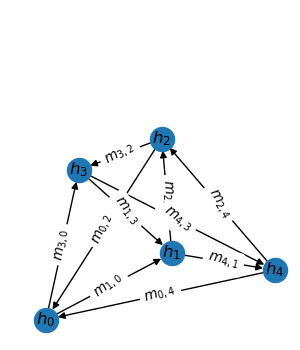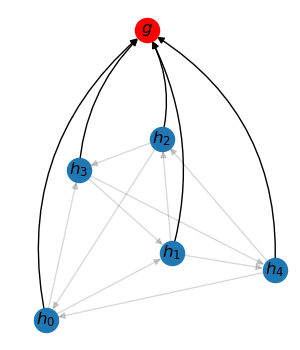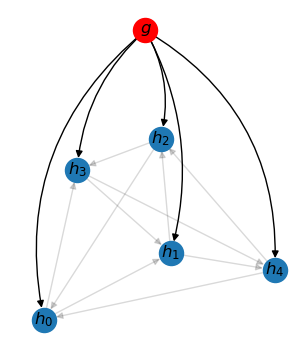# Graph Neural Network Layers#

Modern graph neural networks encode graph structures with message passing layers and readout layers. In some cases, graph-to-node broadcast may also be needed. All these operations can be easily implemented with TorchDrug.Message passingNode-to-graph readoutGraph-to-node broadcast

## Message Passing Layers#

A message passing layer can be described as 3 steps, a message generation step, an aggregation step and a combination step. The $$t$$-th message passing layer performs the following computation

$\begin{split}m_{i,j}^{(t+1)} &= Message^{(t)}(h_i^{(t)}, h_j^{(t)}) \\ u_i^{(t+1)} &= Aggregate^{(t)}(\{m_{i,j}^{(t+1)} \mid j \in N(i)\}) \\ h_i^{(t+1)} &= Combine^{(t)}(h_i^{(t)}, u_i^{(t+1)})\end{split}$

where $$h_i^{(t)}$$ denotes node representations, $$m_{i,j}^{(t)}$$ denotes messages from node $$j$$ to node $$i$$ and $$u_i^{(t)}$$ is the aggregated messages, i.e., updates.

In TorchDrug, these steps are abstracted as three methods, namely message(graph, input), aggregate(graph, message) and combine(input, update).

Here we show an example of a custom message passing for PageRank algorithm. Representing the PageRank value as $$h_i^{(t)}$$, one PageRank iteration is equivalent to the following functions.

$\begin{split}Message^{(t)}(h_i^{(t)}, h_j^{(t)}) &= \frac{h_j^{(t)}}{degree\_in_j} \\ Aggregate^{(t)}(\{m_{i,j} \mid j \in N(i)\}) &= \sum_{j \in N(i)} m_{i,j} \\ Combine^{(t)}(h_i^{(t)}, u_i^{(t+1)}) &= u_i^{(t+1)}\end{split}$

We use the convention that $$degree\_in_j$$ represents the degree of node $$j$$ as the source node of any edge. The corresponding implementation is

from torch_scatter import scatter_add
from torchdrug import layers

class PageRankIteration(layers.MessagePassingBase):

def message(self, graph, input):
node_in = graph.edge_list[:, 0]
message = input[node_in] / graph.degree_in[node_in].unsqueeze(-1)
return message

def aggregate(self, graph, message):
node_out = graph.edge_list[:, 1]
update = scatter_add(node_out, message, dim=0, dim_size=graph.num_node)
return update

def combine(self, input, update):
output = update
return output


Let’s elaborate the functions one by one. In message(), we pick the source nodes of all edges, and compute the messages by dividing the source nodes’ hidden states with their source degrees.

In aggregate(), we collect the messages by their target nodes. This is implemented by scatter_add() operation from PyTorch Scatter. We specify dim_size to be graph.num_node, since there might be isolated nodes in the graph and scatter_add() cannot figure it out from node_in.

The combine() function trivially returns node updates as new node hidden states.

A readout layer collects all node representations in a graph to form a graph representation. Reversely, a broadcast layer sends the graph representation to every node in the graph. For a batch of graphs, these operations can be viewed as message passing on a bipartite graph – one side are original nodes, and the other side are “graph” nodes.

TorchDrug provides effcient primitives to support this kind of message passing. Specifically, node2graph maps node IDs to graph IDs, and edge2graph maps edge IDs to graph IDs.

In this example, we will use the above primitives to compute the variance of node representations as a graph representation. First, we readout the mean of node representations. Second, we broadcast the mean representation to each node to compute the difference. Finally, we readout the mean of the squared difference as the variance.

from torch import nn
from torch_scatter import scatter_mean

class Variance(nn.Module):

def forward(self, graph, input):
mean = scatter_mean(input, graph.node2graph, dim=0, dim_size=graph.batch_size)
diff = input - mean[graph.node2graph]
var = scatter_mean(diff * diff, graph.node2graph, dim=0, dim_size=graph.batch_size)
return var


Notice that node2graph is used for both readout and broadcast. When used in a scatter function, it serves as readout. When used in a conventional indexing, it is equivalent to broadcast.